# Precalculus : Reflections

## Example Questions

### Example Question #2 : Transformations

Reflect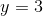across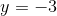, and then across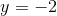. What is the equation of the line after the reflections?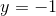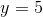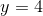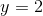Explanation:

The distance between the linesandis 6 units. Therefore, the first reflection should be 6 units below.  Therefore, the first reflection is: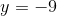The distance betweenandis 7 units.  Therefore, second reflection should be 7 units above.

The result is.

### Example Question #1 : Transformations

Reflectacross.  Reflect again across.  What is the equation of the line after both reflections?Explanation:

The result of reflectingacrosswill become.  Reflecting the equationacrosswill become.

The correct answer is.

### Example Question #23 : Graphing Functions

Ifwas reflected across, then reflected across, and then reflected again across, what is the equation after the three reflections?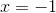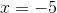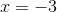Explanation:

First, ifwas reflected across, the new line will be.

Reflecting this lineacrosswill yield.

Reflectingacrosswill yield.

The answer is.

### Example Question #1 : Transformations

Which of these functions has been shifted one unit to the right, three units down, and reflected across the-axis?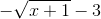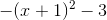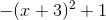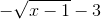Explanation:

So far all of the transformations have come after or inside the function. But what about those that come before the function? This is the basis of reflections which can be made to reflect across the x or the y axis. Since a function takes an x value and returns a y value, the placement of our sign matters. For instance,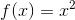is a parabola opening up whose vertex is at the origin. But if one was to place a  -  sign in front of the fucntion like this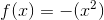, then the function would always return a negative y value. Because of this, a negative sign in front of the function, but not "in" it will reflect over the x axis. This is equivalent to turning the first function,,  a smile, into a frown. Use a graphing utility to visualize this. Now what if you placed a negative sign within the function? This would automatically make all x values negative. And since a function returns a y value and we will always be starting from the negative side of the x axis, this would reflect across the y axis. Use the function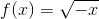to visualize this in a graphing utility.is the correct answer because a negative 1 inside the function (which is within the square root) shifts the function to the right (it is opposite of intuition) one unit and outside the function a negative 3 shifts the function 3 units down. Finally, because our negative sign is outside the function we will always yield negative y values and have thus reflected over the x axis.

### All Precalculus Resources